## Quartic Curve

A general plane quartic curve is a curve of the form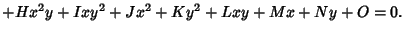(1)
The incidence relations of the 28 bitangents of the general quartic curve can be put into a One-to-One correspondence with the vertices of a particular Polytope in 7-D space (Coxeter 1928, Du Val 1931). This fact is essentially similar to the discovery by Schoutte (1910) that the 27 Solomon's Seal Lines on a Cubic Surface can be connected with a Polytope in 6-D space (Du Val 1931). A similar but less complete relation exists between the tritangent planes of the canonical curve of genus 4 and an 8-D Polytope (Du Val 1931).

The maximum number of Double Points for a nondegenerate quartic curve is three.

A quartic curve of the form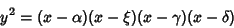(2)

can be written(3)

and so is Cubic in the coordinates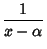(4)(5)

This transformation is a Birational Transformation.Let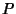andbe the Inflection Points andandthe intersections of the line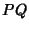with the curve in Figure (a) above. Then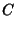(6)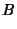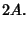(7)

In Figure (b), let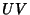be the double tangent, andthe point on the curve whosecoordinate is the average of thecoordinates ofand. Thenand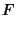(8)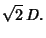(9)

In Figure (c), the tangent atintersects the curve at. Then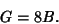(10)

Finally, in Figure (d), the intersections of the tangents atandareand. Then(11)

(Honsberger 1991).

References

Coxeter, H. S. M. The Pure Archimedean Polytopes in Six and Seven Dimensions.'' Proc. Cambridge Phil. Soc. 24, 7-9, 1928.

Du Val, P. On the Directrices of a Set of Points in a Plane.'' Proc. London Math. Soc. Ser. 2 35, 23-74, 1933.

Honsberger, R. More Mathematical Morsels. Washington, DC: Math. Assoc. Amer., pp. 114-118, 1991.

Schoutte, P. H. On the Relation Between the Vertices of a Definite Sixdimensional Polytope and the Lines of a Cubic Surface.'' Proc. Roy. Akad. Acad. Amsterdam 13, 375-383, 1910.